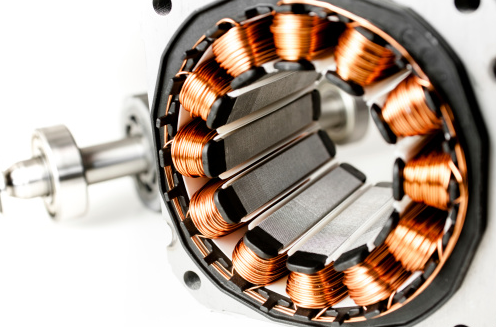## Motor Shaft RPM

A 4 pole induction motor operates with a 0.05 slip, and a line voltage frequency of 30 Hz. What is the motor shaft's rotational speed (rpm)?Hint
The synchronous speed for ac motors is:
$$n_s=120*f/p$$$where $$f$$ is the line voltage frequency in Hz, and $$p$$ is the number of poles. Hint 2 The slip for an induction motor is: $$slip=\frac{(n_s-n)}{n_s}$$$
where $$n$$ is the rotational speed.
The synchronous speed for ac motors is:
$$n_s=120*f/p$$$where $$f$$ is the line voltage frequency in Hz, and $$p$$ is the number of poles. For a 4 pole motor operating on 30 Hz voltage: $$n_s=120*30/4=900\:rpm$$$
The slip for an induction motor is:
$$slip=\frac{(n_s-n)}{n_s}$$$where $$n$$ is the rotational speed. $$0.05=\frac{(900rpm-n)}{900rpm}$$$
Solving for $$n$$ :
$$n=900rpm-0.05(900rpm)=900rpm-45rpm=855\:rpm$$\$
855 rpm